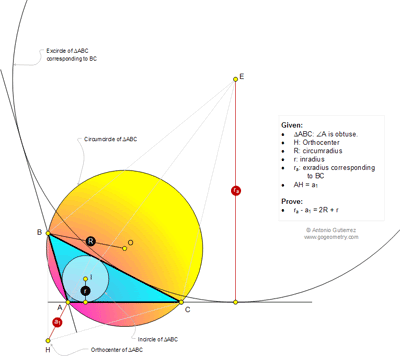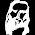## Thursday, December 4, 2014

Level: Mathematics Education, High School, Honors Geometry, College.

Click the diagram below to enlarge it.#### 1 comment:

1.Since ΔABC and ΔHBC have the same circumradius,
a₁ = 2R cosH = −2R cosA
r = 4R sin(A/2) sin(B/2) sin(C/2)
r₁ = 4R sin(A/2) cos(B/2) cos(C/2)

r₁ − r = 4R sin(A/2) cos(B/2 + C/2)
= 4R sin²(A/2) = 2R(1−cosA) = 2R + a₁
a₁ + r₁ − r = 2R
r₁ − a₁ = 2R + r